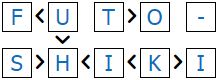Futoshiki

Futoshiki also known as "More or Less" is a logic puzzle with simple rules and challenging solutions.

The rules are simple.
You have to fill the grid with numbers so that:
- The numbers are from 1 to the size of the grid i.e. 1 to 5 for a 5x5 puzzle.
- Each row and column must contain only one instance of each number.
- The numbers should satisfy the comparison signs - less than or greater than.
- For bigger puzzles the letters (A,B,C etc.) follow after the number 9.

Video TutorialFutoshiki 4x4 Easy Puzzle ID: 5,351,940

Share Share